Latest Banking jobs   »

# Quantitative Aptitude Quiz For IBPS PO Prelims 2022- 19th September

Q1. Sum of the upstream speed and downstream speed of a boat is 48 km/h. If speed of current is 80% less than speed of boat in still water, then find time taken by boat to travel 288 km in downstream.
(a) 7 hours
(b) 10 hours
(c) 5 hours
(d) 2 hours
(e) 15 hours

Quantitative Aptitude Quiz For IBPS PO Prelims 2022- 19th September check in hindi

Q2. Respective ratio of present ages of A and B is 12:5. After 8 years, A’s age will become twice of B’s age, find present ages of A?
(a) 52 years
(b) 56 years
(c) 44 years
(d) 48 years
(e) 40 years

Q3. A can do a work in 18 days while B can do the same work in 15 days. Both of them started the work together but A left the work after 3 days and remaining work completed by B and C in 3.8 days. Find the time taken by C to completed the whole work.
(a) 12 days
(b) 15 days
(c) 5 days
(d) 14 days
(e) 10 days

Q4. A and P started a business by investing Rs.5000 and 8000 respectively. After 4 months A withdrew 50% of his investment. If at the end of the year, profit share of P is Rs.8400, then find the profit share of A.
(a) Rs. 2500
(b) Rs. 3000
(c) Rs. 4000
(d) Rs. 3500
(e) Rs. 5500

Q5. If the length of each edge of a cube is √8 cm, then find the lateral surface area of the cube.
(a) 32 cm²
(b) 16 cm²
(c) 64 cm²
(d) 128 cm²
(e) 4 cm²

Q6. A shopkeeper sells an article at a profit of 10%. Had he bought it for 5% less and sold it for 240 Rs. More, then he would have gained 20% profit. Find the cost price of the article?
(a)Rs 5000
(b) Rs 8000
(c) Rs 6000
(d) Rs 7000
(e) Rs 4000

Q7. P started a business with a capital of Rs. 6000, after four months Q joined him with a capital of Rs. (P+2000). If at the end of the year they get equal share in profit, then find the capital invested by Q?
(a) 8000 Rs.
(b) 9000 Rs.
(c) 6000 Rs.
(d) 5000 Rs.
(e) 7500 Rs.

Q8. Train A crosses a 98 meters long tunnel in 24 seconds. Train B having same length as train A crosses a pole in 12 seconds. If speed of train A is 20% more than speed of train B, then find length (in meters) of train A?
(a) 80
(b) 65
(c) 70
(d) 75
(e) 90

Q9. A and B together can complete a piece of work in 16 days, while B and C together can complete the same work in 24 days. If A and C together can complete the work in 48 days, then find in how many days all three together can complete half of the work?
(a) 8 days
(b) 10 days
(c) 6 days
(d) 12 days
(e) 16 days

Q10. 60 % of their respective number of people are post graduate from two towns P and Q. If the sum of total graduates from these two towns is 8400, then find the sum of total people in the both towns (Total people in any town = graduate + post graduate)?
(a) 21000
(b) 18000
(c) 16000
(d) 14000
(e) 12000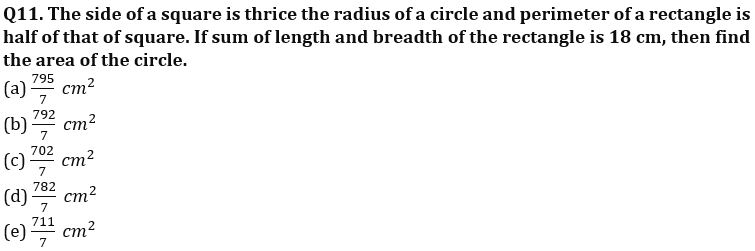Q12. Rekha invested same amount Rs. P in S.I. and C.I. If difference between S.I. and C.I. earned after 2 years at same rate of 18% is Rs.810, then find the value of P.
(a)25000
(b)28000
(c)22000
(d)24000
(e)29500

Q13. Aarti bought 15 kg of mangoes for Rs.450, out of which 3 kg rotten. At what price (per kg) should she sell the remaining mangoes to earn a profit 20%.
(a) Rs.42
(b) Rs.48
(c) Rs.40
(d) Rs.45
(e) Rs.38

Q14. Income of Chris is Rs.25000, out of which he saves 28%. If his expenditure decreases by 35% while his income remains the same, then find the ratio of his new savings to old savings.
(a) 8:5
(b) 19:10
(c) 12:7
(d) 17:9
(e) 15:8

Q15. Ratio of age of A four years ago from now and age of B six years hence from now is 2: 5 respectively. If the ratio of present age of B and C is 19:15 respectively and present age of A is 14 years, then find the age of C five year hence.
(a) 20 years
(b) 22 years
(c) 15 years
(d) 10 years
(e) 25 years

Solutions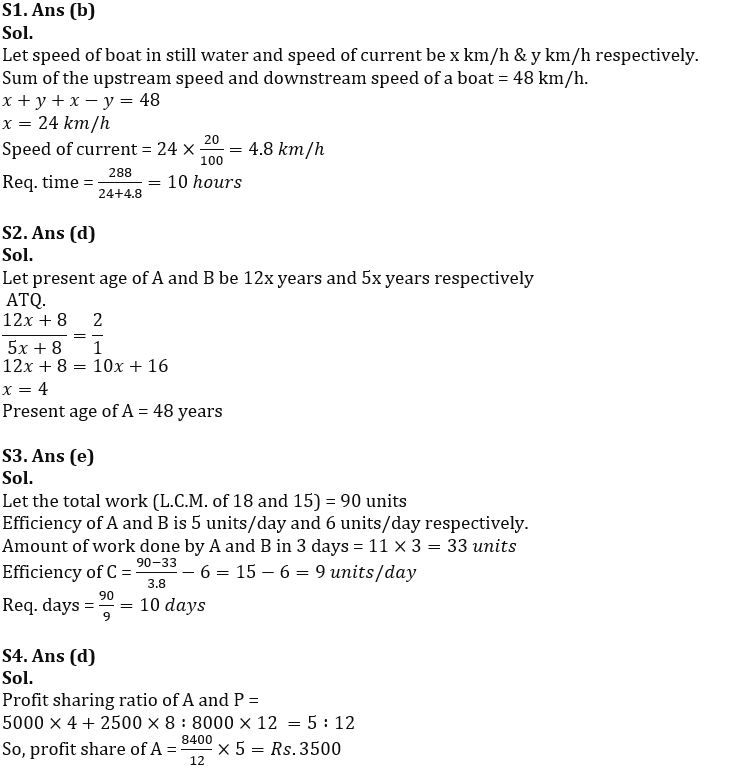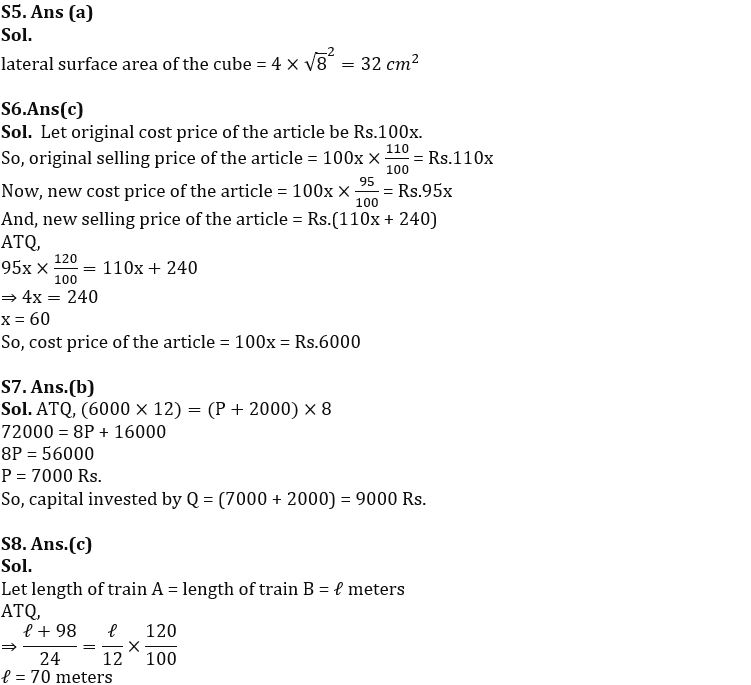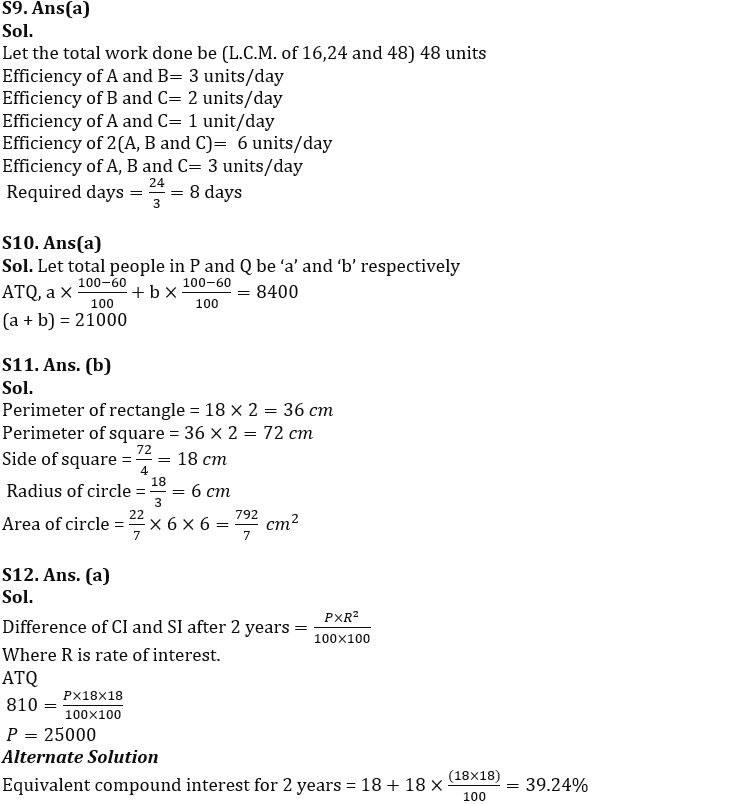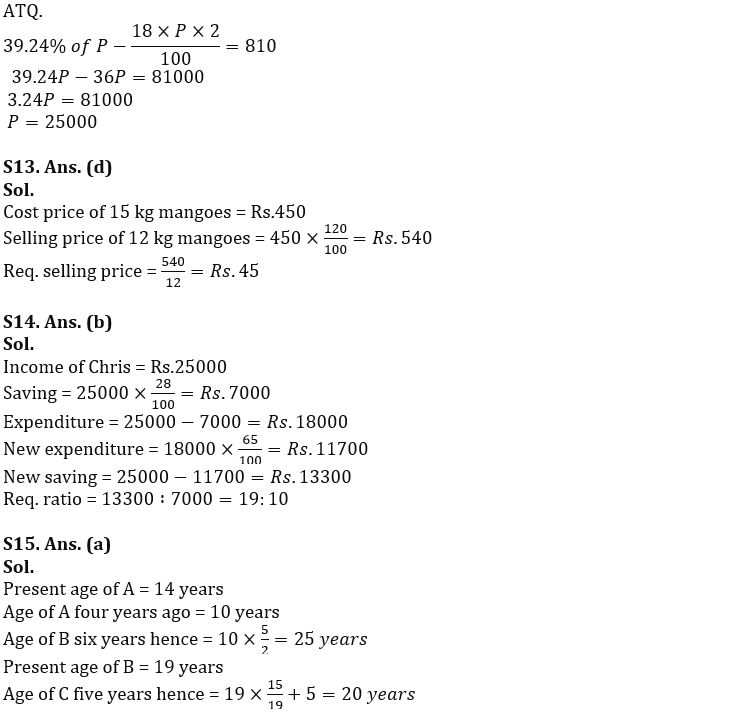#### Congratulations!Download Hindu Review of October 2021: Free PDF